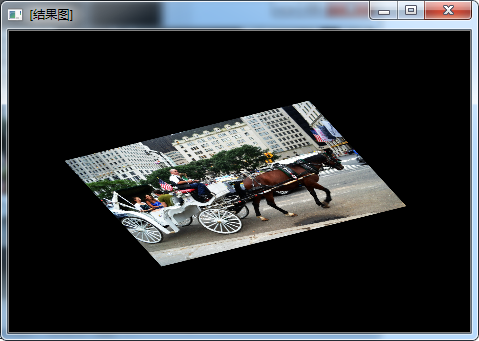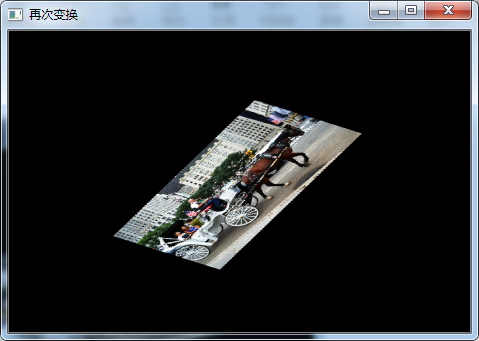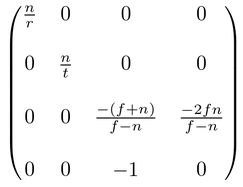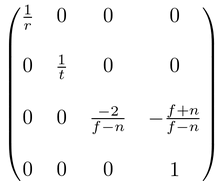• 求变换矩阵
千次阅读
2021-09-14 07:19:50

### 目录

SVD是点云配准中最常用计算方法之一，看到网上有很多写实现过程的代码，但是，，，，，。自己去搜一下，再结合算法原理理解一下就知道代码是否正确或完整了！！！！

# 一、算法原理

## 2、详细过程

见：

更多相关内容
• //仿射变换，根据点求变换矩阵以及根据角度和缩放因子求变换矩阵 Mat mapx; Mat mapy; Mat src; Mat dst; int main() { //1.原图像。 src = imread("马车2.jpg"); resize(src, src, Size(), 0.1, 0.1); imshow...


//仿射变换，根据点求变换矩阵以及根据角度和缩放因子求变换矩阵

Mat mapx;
Mat mapy;
Mat src;
Mat dst;
int main()
{
//1.原图像。
resize(src, src, Size(), 0.1, 0.1);
imshow("效果图",src);

//2.确定两组点，每组点3个。
Point2f src_triangle;
src_triangle = Point2f(0.,0.);
src_triangle = Point2f(0.,static_cast<float>(src.rows-1));
src_triangle = Point2f(static_cast<float>(src.cols-1),0.);
Point2f dst_triangle;
dst_triangle = Point2f(0.12*src.cols,0.43*src.rows);
dst_triangle = Point2f(0.33*src.cols,0.78*src.rows);
dst_triangle = Point2f(0.65*src.cols,0.23*src.rows);
//3.从两组点来计算变换矩阵。//getAffine放射矩阵。
Mat fist_trans;
fist_trans.create(2,3,CV_32FC1);
fist_trans=getAffineTransform(src_triangle,dst_triangle);
//3.1计算转换后的图像
dst.create(src.rows,src.cols,src.type());
warpAffine(src,dst,fist_trans,src.size());
imshow(window,dst);
//4.再将变换后图像再次变换一个角度，和一个缩放。
float theta = 30.0;
float scale = 0.78;

//5.根据这个角度和缩放计算第二次变换的变换矩阵。getRotiation//仿射矩阵。
Mat second_mtrax;
second_mtrax.create(2,3,CV_32FC1);
second_mtrax=getRotationMatrix2D(Point2f(dst.cols/2.0,dst.rows/2.0),theta,scale);

//6.应用变换矩阵进行变换。warpaffine//仿射变换。
Mat dst2;
warpAffine(dst,dst2,second_mtrax,dst.size());
imshow("再次变换",dst2);

waitKey(0);
return 0;

}展开全文opencv 计算机视觉 仿射变换
• MATLAB 函数查找以方程形式给出的线性变换矩阵表示。 详细阅读评论部分。matlab
• 基于OpenCV的二维矩阵刚性变换（旋转，平移），已知两点集，求解R&T变换矩阵，其中自定义接口，已经赋初值，可以更改调用
• ## 变换矩阵

千次阅读 2018-10-27 16:58:25
1、变换矩阵 变换矩阵可以分解为缩放，旋转，平移矩阵的乘积： M = T * R * S - 右手坐标系 当均匀缩放时，旋转和缩放可以交换顺序 缩放和平移不可以交换顺序 2、子坐标系与父坐标系 由在父坐标系中的坐标位置...

1、变换矩阵

变换矩阵可以分解为缩放，旋转，平移矩阵的乘积：

M = T * R * S - 右手坐标系

当均匀缩放时，旋转和缩放可以交换顺序

缩放和平移不可以交换顺序

2、子坐标系与父坐标系

由在父坐标系中的坐标位置P，和三根轴X，Y，Z可定义一个子标系，按列构成一个变换矩阵[X,Y,Z,P]，这个矩阵构成由子标系变换到父坐标系的变换矩阵。DX里面需要转置一下。即按行构造。

一个例子是视图矩阵的构建，子空间由视点和三根正交轴定义，视图矩阵则是由它们构成的列矩阵的仿射求逆-由世界变换到视图空间。

另一个例子世界变换矩阵，它由新模型坐标系的位置和三根轴【定义在世界空间】按列构成。即由模型空间变换到世界空间。初始情况模型空间与世界空间是对齐的。

3、投影矩阵-OpenGL

透视投影矩阵：可以知道投影变换后的w=-Ze，即在视图空间的深度

在投影面上的坐标Xp, Yp为：Xp=n*Xe/-Ze=Xclip/w*r，Yp=n*Ye/-Ze=Yclip/w*t

投影后Ze从[-n,-f]线性映射到[-n,f]，除以w后进一步非线性映射到[-1,1]

透视投影除以w后，视景体的八个角点【视图空间坐标】分别映射到NDC空间中的八个角点，如[r,t,-n,1]映射到[1,1,-1,1]，[f*r/n,f*t/n,-f,1]映射到[1,1,1,1]

正交投影矩阵：变换后w=1，Xe，Ye，Ze被线性映射到-1~1，而透视投影是非线性映射，因而有Z-Fighting问题。

4.应用例子：从深度图重建位置

展开全文图形 变换矩阵
• ## 求若当标准型的变换矩阵

千次阅读 多人点赞 2020-11-19 14:52:29
但在这里掐指一算就知道了，因为只有 2 是二重根 下面要求变换矩阵 P P P 使得： A = P J P − 1 ⇔ A P = P J ⇔ A [ p 1 p 2 p 3 ] = [ p 1 p 2 p 3 ] [ 2 0 0 1 2 0 0 0 4 ] ⇔ { A p 1 = 2 p 1 + p 2 A p 2 = 2 ...

已知
A = [ 2 0 0 1 4 0 1 0 2 ] A = \begin{bmatrix} 2 & 0 &0 \\ 1 & 4 & 0\\ 1 & 0 & 2 \end{bmatrix}
特征多项式为：
∣ λ I − A ∣ = ∣ λ − 2 0 0 − 1 λ − 4 0 − 1 0 λ − 2 ∣ = ( λ − 2 ) 2 ( λ − 4 ) = 0 |\lambda I - A| = \begin{vmatrix} \lambda -2 & 0 &0 \\ -1 & \lambda -4 & 0\\ -1 & 0 & \lambda -2 \end{vmatrix} = (\lambda - 2)^2(\lambda -4)=0
求出特征值： λ = 2 (二重) , 4 \lambda = 2\text{(二重)}, 4 .

但是显然 A A 不会相似于对角矩阵:
[ 2 0 0 0 4 0 0 0 2 ] \begin{bmatrix} 2 & 0 &0 \\ 0 & 4 & 0\\ 0 & 0 & 2 \end{bmatrix}
因而只能是相似于若当标准型：
J = [ 2 0 0 1 2 0 0 0 4 ] J = \begin{bmatrix} 2 & 0 &0 \\ 1 & 2 & 0\\ 0 & 0 & 4 \end{bmatrix}
注意：若当标准型的标准求法需要用到 λ \lambda -多项式(或 λ \lambda -矩阵)，参见高等代数教材。但在这里掐指一算就知道了，因为只有 2 是二重根

下面要求变换矩阵 P P 使得：
A = P J P − 1 ⇔ A P = P J ⇔ A [ p 1 p 2 p 3 ] = [ p 1 p 2 p 3 ] [ 2 0 0 1 2 0 0 0 4 ] ⇔ { A p 1 = 2 p 1 + p 2 A p 2 = 2 p 2 A p 3 = 4 p 3 ⇔ { ( A − 2 I ) p 1 = p 2 ⇒ ( A − 2 I ) 2 p 1 = 0 ( A − 2 I ) p 2 = 0 ( A − 4 I ) p 3 = 0 \begin{aligned} &A = PJP^{-1} \\ \Leftrightarrow& AP = PJ \\ \Leftrightarrow& A \begin{bmatrix}p_1 & p_2 & p_3\end{bmatrix} = \begin{bmatrix}p_1 & p_2 & p_3\end{bmatrix} \begin{bmatrix} 2 & 0 &0 \\ 1 & 2 & 0\\ 0 & 0 & 4 \end{bmatrix} \\ \Leftrightarrow& \begin{cases} A p_1 = 2p_1 + p_2 \\ A p_2 = 2p_2 \\ A p_3 = 4p_3 \end{cases} \\ \Leftrightarrow& \begin{cases} (A - 2I) p_1 = p_2 \Rightarrow (A - 2I)^2 p_1 = 0\\ (A-2I) p_2 = 0 \\ (A-4I) p_3 = 0 \end{cases} \end{aligned}
p 3 p_3 A − 4 I A-4I 的核空间，很好求:
( A − 4 I ) p 3 = [ − 2 0 0 1 0 0 1 0 − 2 ] p 3 = 0 ⇒ p 3 = [ 0 1 0 ] (A-4I)p_3 = \begin{bmatrix} -2 & 0 &0 \\ 1 & 0 & 0\\ 1 & 0 & -2 \end{bmatrix} p_3 = 0 \Rightarrow p_3= \begin{bmatrix} 0 \\ 1 \\ 0 \end{bmatrix}
p 2 p_2 A − 2 I A-2I 的核空间； p 1 p_1 ( A − 2 I ) 2 (A-2I)^2 的核空间，但是不在 A − 2 I A-2I 的核空间

这该怎么求呢？

先求 p 1 p_1 :
( A − 2 I ) 2 p 1 = [ 0 0 0 1 2 0 1 0 0 ] 2 p 1 = [ 0 0 0 2 4 0 0 0 0 ] p 1 = 0 (A-2I)^2p_1 = \begin{bmatrix} 0 & 0 &0 \\ 1 &2 & 0\\ 1 & 0 &0 \end{bmatrix}^2 p_1 = \begin{bmatrix} 0 & 0 &0 \\ 2 &4 & 0\\ 0 & 0 &0 \end{bmatrix} p_1 =0 ⇒ p 1 = [ 0 0 1 ] 或者 [ − 2 1 0 ] \Rightarrow p_1= \begin{bmatrix} 0 \\ 0 \\ 1 \end{bmatrix} \text{或者} \begin{bmatrix} -2 \\ 1 \\ 0 \end{bmatrix}
由于前者 [ 0 0 1 ] \displaystyle \begin{bmatrix}0 \\0 \\1 \end{bmatrix} A − 2 I A-2I 的核空间，故舍弃，所以 p 1 = [ − 2 1 0 ] \displaystyle p_1 = \begin{bmatrix} -2 \\1 \\0 \end{bmatrix}
所以
p 2 = ( A − 2 I ) p 1 = [ 0 0 0 1 2 0 1 0 0 ] [ − 2 1 0 ] = [ 0 0 − 2 ] p_2 = (A-2I)p_1 = \begin{bmatrix} 0 & 0 &0 \\ 1 &2 & 0 \\ 1 & 0 &0 \end{bmatrix} \begin{bmatrix} -2 \\1 \\0 \end{bmatrix} = \begin{bmatrix} 0 \\0 \\-2 \end{bmatrix}
至此， P P 矩阵求出

可以验证：
A P = [ 2 0 0 1 4 0 1 0 2 ] [ − 2 0 0 1 0 1 0 − 2 0 ] = [ − 4 0 0 2 0 4 − 2 − 4 0 ] AP = \begin{bmatrix} 2 & 0 &0 \\ 1 & 4 & 0\\ 1 & 0 & 2 \end{bmatrix} \begin{bmatrix} -2 & 0 &0 \\ 1 & 0 & 1\\ 0 & -2 & 0 \end{bmatrix} = \begin{bmatrix} -4 & 0 &0 \\ 2 & 0 & 4\\ -2 & -4 & 0 \end{bmatrix}
P J = [ − 2 0 0 1 0 1 0 − 2 0 ] [ 2 0 0 1 2 0 0 0 4 ] = [ − 4 0 0 2 0 4 − 2 − 4 0 ] = A P PJ = \begin{bmatrix} -2 & 0 &0 \\ 1 & 0 & 1\\ 0 & -2 & 0 \end{bmatrix} \begin{bmatrix} 2 & 0 &0 \\ 1 & 2 & 0\\ 0 & 0& 4 \end{bmatrix} = \begin{bmatrix} -4 & 0 &0 \\ 2 & 0 & 4\\ -2 & -4 & 0 \end{bmatrix} = AP
A = P J P − 1 A = PJP^{-1}

展开全文若当标准型 变换矩阵
• 已知不同坐标系的多个坐标点的坐标，两个坐标系的转换矩阵,是matlab写的程序，三维坐标的。 做机器人视觉导航时用到的这个转换。
• 只要算出变换矩阵，就可以算出A坐标系的一个点P在坐标系B里的对应点坐标，即 R为3x3的转换矩阵， t 为3x1的位移变换向量，这里点坐标均为3x1的列向量（非齐次形式，齐次形式下为4x1列向量，多出的一个元素值补1而已...opencv
• 我们需要计算出一个变换矩阵，从而在已知物体在旧坐标系的坐标的情况下，通过这个变换矩阵获得物体在新坐标系的坐标。这篇文章讲述的是一个获得这个变化矩阵的方法。这个方法是在参考资料里的视频学到的，有兴趣的...计算机图形 坐标变换
• `python import numpy as np def rotation_matrix_from_vectors(vec1, vec2): """ Find the rotation matrix that aligns vec1 to vec2 :param vec1: A 3d "source" vector :param vec2: A 3d "destination" ...机器学习
• 提高压缩比，DCT快速变换的限制较少，该转换技术只需要在实数域上就可以实现
• ## OpenCV 获取变换矩阵

千次阅读 2020-01-03 17:15:09
1. 通过点和角度，获取仿射变换矩阵，进而获取矩阵变换后的图像 Mat dstImage; Mat affine_matrix = getRotationMatrix2D(center, angle, 1.0); warpAffine(img, dstImage, affine_matrix, img.size()); 2. 通过...
• 过渡矩阵的方法 -个由基a, a,., a,到B, .,… β.的过渡矩阵P, 一般采用下列方法: (1)定义法.将βi，i=1,2…,n, 在基ai下的坐标逐个出，按列写成一一个n阶矩阵，即为过渡矩阵P: 函数R[x]_5旧基为B1={1，x, x2, ...
• 文章目录旋转矩阵平移变换矩阵与齐次坐标TF中发布坐标变换 在空间中表示两个物体之间的位姿关系，通常可以分解为一个纯平移和一个纯旋转。也称为一个欧式变换。 旋转矩阵 这里介绍一种用旋转矩阵表示旋转的方式。...
• 实现方法：棕熊的肚皮1 公式推导：棕熊的肚皮2 转载过来公式全是乱的！
• 输入是两帧的位姿放入qw,qc,tw,tc中，记住qw，qc里的第一个参数是TUM GroundTruth里位姿的最后一项，输出是两帧的变换矩阵T，下载就能用。
• ret_R, ret_t = rigid_transform_3D(A, B) # SVD分解计算变换矩阵 A2 = (ret_R*A.T) + tile(ret_t, (1, n)) A2 = A2.T # 与刚开始随机生成的变换矩阵进行比较，计算误差。 err = A2-B err = multiply(err, err...python
• 解构变换矩阵 给定一个转换的复合矩阵，关于组成该转换的任何单个转换的信息就会丢失。 我们如果有一个复合矩阵，怎么能使其分解为TRS三个矩阵呢？即如何完成下述变化： 其中M是给定的变换矩阵，T是平移矩阵，...
• matlab开发-Haar小波变换矩阵化。创建Haar小波变换矩阵
• 【OpenGL】二十、OpenGL 矩阵变换 ( 矩阵缩放变换 | 矩阵旋转变换 | 矩阵平移变换 ) https://hanshuliang.blog.csdn.net/article/details/112859256 博客源码 ( 该源码是 Windows 桌面程序 , 使用 Visual Studio ...OpenGL
• ####问题：假定下图中的A坐标系是世界坐标系，我们想出目标坐标系B到世界坐标系的旋转矩阵。 从B到A的变换既有旋转也有平移。从B到A的变换矩阵是一个4x4的矩阵算子。 B到A的旋转矩阵的定义式： 其中 ...
• ## 详解坐标变换矩阵

万次阅读 多人点赞 2021-12-27 22:54:28
这是本人第一次在CSDN发文，本文详细总结坐标变换矩阵。 一、何为坐标变换矩阵 首先要回答一个问题，何为坐标变换矩阵呢？在以上所举例的坐标系变换的语境下， “点不变，坐标系进行变换。” 高中就接触到点的...
• Matlab软件求矩阵的特征值，进行矩阵的初等变换；讨论向量组的线性相关性等运算。
• 只要算出变换矩阵，就可以算出A坐标系的一个点P在坐标系B里的对应点坐标，即 T为3x3的转换矩阵， t 为3x1的位移变换向量，这里点坐标均为3x1的列向量（非齐次形式，齐次形式下为4x1列向量，多出的一个元素值补1...
• 只要能算出变换矩阵，就可以算出A坐标系的一个点P在坐标系B里的对应点坐标，即P(B)=T*P(A)+t,T为3x3的转换矩阵，t为3x1的位移变换向量，点坐标均为3x1的列向量。 只要给定至少3个点，就能计算出T和t，一般来说点越...
• 三维坐标变换矩阵的推导过程 在3D计算机图形学中，我们经常需要使用多个坐标系，因此我们需要知道如何从一个坐标系转到另一个坐标系。在3D计算机图形学中，点(Point)和向量(Vector)的变换是不同的，所以需要分别...
• 变换形式如下，a0, a1, a2, b0, b1,b2是对应2*3变换矩阵的几个值。 针对图像而言，变换矩阵和图像之间关系为：dst_img=M*src_img 仿射变换有如下几种变换形式：平移、旋转、放缩、剪切、翻转。 为了涵盖平...仿射变换 变换矩阵
• ## 仿射变换矩阵

千次阅读 2022-04-19 10:58:39
首先总结而言：仿射变换矩阵实际上就是：平移向量+旋转变换组合而成，可以同时实现旋转，缩放，平移等空间变换。 Eigen库中，仿射变换矩阵的大致用法为： 创建Eigen::Affine3f 对象a。 创建类型为Eigen::Translation......